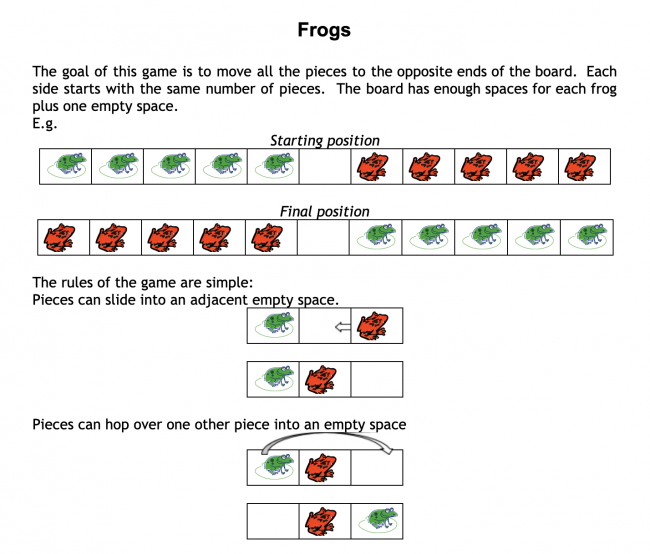# Hopping Frogs

Preview:Students investigate the minimum number of moves required to complete the game and then find a rule which would enable them to state how many moves would be required to play the game with any number of frogs on each side.

Project
Big Idea:
Grade 8: Number represents, describes, and compares the quantities of ratios, rates, and percents.
Curricular Competency:
Reasoning and analyzing: Use reasoning to explore and make connections
Reasoning and analyzing: Model mathematics in contextualized experiences
Understanding and solving: Develop, demonstrate, and apply mathematical understanding through play, inquiry, and problem solving
Understanding and solving: Develop and use multiple strategies to engage in problem solving
Communicating and representing: Communicate mathematical thinking in many ways
Communicating and representing: Explain and justify mathematical ideas and decisions